### Home > A2C > Chapter Ch9 > Lesson 9.2.3 > Problem9-105

9-105.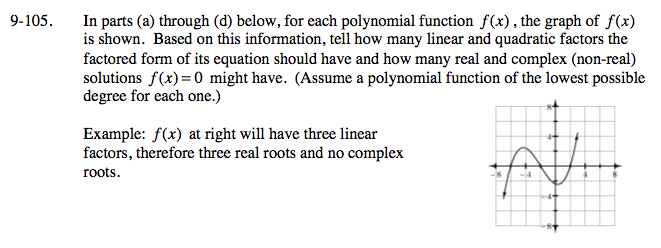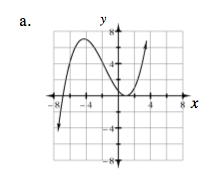There will be three linear factors (one is repeated), so the function will have two unique real roots and no complex roots.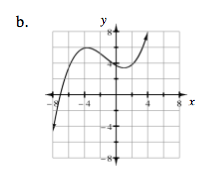There will be one linear factor and one quadratic factor, so the function will have one real root and two complex roots.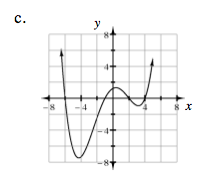There will be four linear factors, so the function will have four real roots.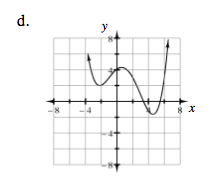There will be two linear factors and one quadratic factor, so the function will have two real roots and two complex roots.